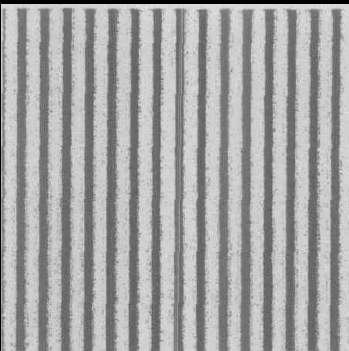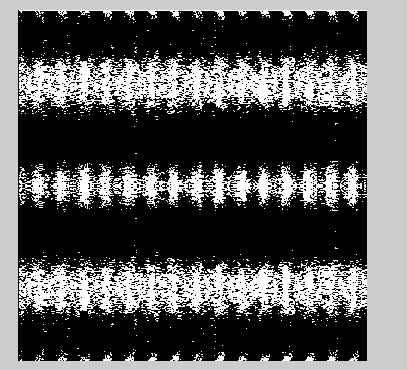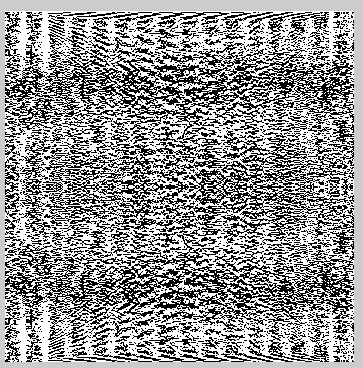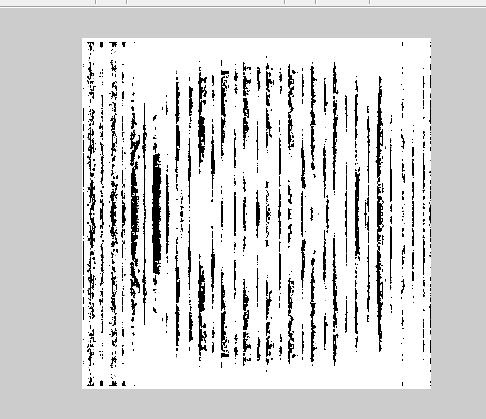2017-04-12 04:57

# 有关结构光三维重建提取相位

5

fs=0.065;
I=rgb2gray(Q);
figure(1);
% subplot(3,3,1);
imshow(I);
G=rgb2gray(R);
figure(2);
% subplot(3,3,2);
imshow(G);
FW=fft(I);
% FW1=abs(FW);
figure(3);
% subplot(3,3,3);
imshow(FW);
GW=fft(G);
% GW1=abs(GW);
figure(4);
% subplot(3,3,4);
imshow(GW);
Fb=FW-GW;
TW=ifft(Fb);
figure(5);imshow(Fb);
QW=ifft(Fb);
figure(6);imshow(QW);%对频域相减后空域
mag=abs(Fb);%求解相减后幅值
f=(0:351-1)*fs/351;%横坐标频率的表达式为f=(0:M-1)*Fs/M;
% TW=ifft(mag);
% figure(7);
plot(f,mag);%做频谱图
% subplot(3,3,5);
figure(8);
imshow(TW);
% W=G-I;
% subplot(3,3,6);imshow(W);

% % 正弦光栅
% clc;close all;clear all;
% f=0.065;%频率为0.125
% for i=1:800
% for j=1:800
% Img(i,j)=0.5+0.5*cos(2*pi*f*j);
% end
% end
% figure(1);imshow(Img,[]);• 点赞
• 写回答
• 关注问题
• 收藏
• 复制链接分享
• 邀请回答

#### 2条回答

• 我要做的是通过一个黑白条纹对一个物体进行照射，条纹频率代码有，然后先从图片中提取相关的相位，但是我只能通过MATLAB求的该图片的频谱，有大神解答一下吗？看看代码哪里要修改

点赞 评论 复制链接分享
• 点赞 评论 复制链接分享